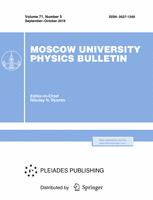Faculty of Physics
M.V.Lomonosov Moscow State University

# The observed Higgs boson as a scalar of indefinite CP-parity of minimal supersymmetry

## M. N. Dubinin, E. Yu. Fedotova

### Memoirs of the Faculty of Physics 2023. N 4.

• Article
Annotation

The Higgs sector of minimal supersymmetry with explicitly CP violation is considered under the assumption that the lightest neutral Higgs boson without definite CP parity is associated with the observed scalar. The analysis of the alignment limit leads to two possibilities for its realization: 1) the condition $\beta-\alpha \simeq \pi/2$ , known in the CP-conserving limit, is supplemented by $c_1 \simeq 0$, $k_1 \xi_1^{I} =1$; 2) the masses of the Higgs bosons are not decoupled and of the order of the electroweak scale, and $m_H^2 \simeq m_{h_1}^2$, $c_2 \simeq -c_1 \tg (\beta - \alpha)$, $k_1 \xi^{\rm II}_1 \xi^{\rm II}_2 \xi^{\rm II}_3 =1$. CP-violating effects in the alignment limit and the values of CP mixing angle $\alpha_{\rm cp}$ are discussed.

Approved: 2023 August 1
PACS:
14.80.Cp Non-standard-model Higgs bosons
11.30.Pb Supersymmetry
11.30.-j Symmetry and conservation laws
11.15.-q Gauge field theories
Authors
M. N. Dubinin, E. Yu. Fedotova
$^1$\
$^2$M.V.Lomonosov Moscow State University, Skobeltsyn Institute of Nuclear Physics (SINP MSU)### Moscow University Physics Bulletin### Science News of the Faculty of Physics, Lomonosov Moscow State University

This new information publication, which is intended to convey to the staff, students and graduate students, faculty colleagues and partners of the main achievements of scientists and scientific information on the events in the life of university physicists.